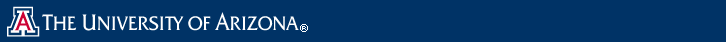# Validating Cost Models

Overview

Algorithms are generally characterized by their computational complexity in terms of asymptotic complexity, in order notation. Such a characterization can be proven by a mathematical analysis. This analysis identifies the most frequent operation and asserts that the complexity is related, as the size of the input goes to infinity, to the number of times that operation is evaluated. So merge sort has worst-case complexity O(n lgn) in the number of comparisons, as the number of items being sorted, n, goes to infinity. By its very construction, asymptotic complexity cannot be proven (or disproven) by an experiment, and so is a mathematical theorem, not a scientific theory. Algorithmic ergalics adopts the scientific perspective, augmenting the mathematical perspective of asymptotic complexity, by attempting to determine a cost formula, which states the execution time or space of an implementation of an algorithm based on a number of parameters, including input size but also including type of processor, speed of main memory, etc., with the goal being to scientifically validate the cost formula or interesting statements about the formula.

People
Faculty